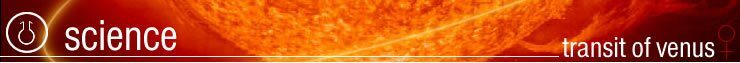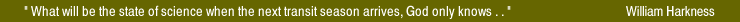home | what is it ?| Horrocks| news| events| observing| science| on the day| quiz| vt-2004| downloads| links|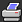#### The Scientific Significance

In this section we cover:

• What can be measured during a Transit of Venus
• The importance of those measurements for the whole of astronomy
• The mathematics required to go from a measurement to a scientific result

One of the fundamental aims of astrophysics over the last 500 years has been the measurement of distance. To measure distances we need some sort of "metre rule" we can lay out across interplanetary, interstellar and intergalactic distances. The basic "metre stick" of astronomy is the distance between the Earth and the Sun, appropriately called the Astronomical Unit or just au.This can be determined by observing the transit of a planet across the face of the Sun. Only planets inside the Earth's orbit can manage this. Thus the transit of Venus provides an opportunity to measure the distance to the Sun. Such a measurement in 1639 represented the beginning of astrophysics in England.

#### The Astronomical Unit

The astronomical unit is tiny on the scale of the Universe:

• The distance between the Sun and the Earth is 1 au = 150 million km.
• The distance to the nearest star is about 250,000 au.
• The diameter of the Galaxy is about 10,000 million au.
• The size of the Universe is about 1000 million million au.

Thus measuring interstellar and intergalactic distances with the au is akin to measuring the distance to the Moon by laying millions of pennies end-to-end. Why then is it so important? For the simple reason that we can measure it! Every other means of measuring astronomical distances give only relative values.

#### Relative distances

The means of measuring astronomical distances include:

Trigonometry
Measuring the angles through which stars and planets move on the sky and working out the paths they must be following.
Standard candles

Measuring the apparent brightness of stars or galaxies and comparing with the intrinsic brightness.

Standard sizes

Measuring the apparent sizes of galaxies or galaxy clusters and comparing with intrinsic sizes.

You will see from this that we can gradually move outwards to ever more distant objects, and in fact standard candles and standard sizes can both be used out to tremendous distances. However, all these methods give only relative distances. We need to determine an absolute distance that can be related to these relative distances.

#### Parallax

Move your head from side to side, and objects in the foreground will appear to move relative to objects in the background. That is parallax. In astronomy, the entire Earth moves from one side of the Sun to the other over the course of 6 months. This results in the nearest stars (in the foreground) appearing to move relative to more distant stars (in the background). In fact this motion is measurable but very small, so small that this was our first solid clue that the stars were much further away than the planets. As the Earth is 1 au from the Sun, it moves by 2 au "side to side" over 6 months. Simple geometry leads to the distance in au. But until we measure the au we are still left with a relative distance, such as the nearest star being 250,000 au from the Sun. This is called calibration.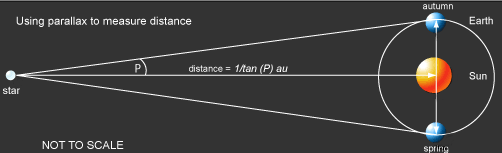#### Using a transit

NEVER OBSERVE THE SUN WITH THE NAKED EYE OR THROUGH A TELESCOPE, CAMERA OR OTHER OPTICAL DEVICE

When Venus is in transit across the Sun, observations from several places on the Earth allow us to measure the distance to Venus in km. As we already know the distance between the Sun and Venus in au, we have calibrated the Astronomical Unit.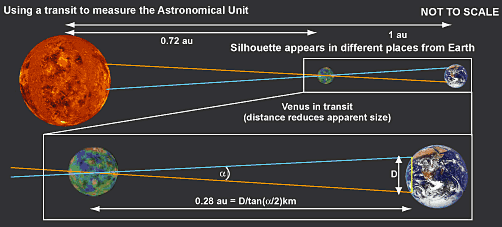The process is one of triangulation. Because the Sun appears to have a diameter of around 30 times Venus, the silhouette seen from different places on Earth appears against different points on the Sun. This is parallax taking effect again. Hence if the transit is observed from places a know distance apart in km, simple geometry gives the distance to Venus in km. As we know that Venus is 0.72 au from the Sun, during the transit it must be 0.28 au from the Earth.

#### Timing the transit

Of course there is always a complication. How do people hundreds of km apart compare the position of silhouette? The answer is in three parts:

• Decide on a particular stage of the transit that everyone will observe
• Record the exact time of that event
• Record the exact position of that event on the Sun

Today this can be done essentially automatically. A computer controlled telescope and camera can take a series of images, and stamp each with an exact time. In the past this was not possible. Instead astronomers agreed well before hand on particular points in the transit to observe , and then went off to their widely separated observing locations with accurate watches. The events had to be easily identifiable, and the start and end of the transit were chosen. In fact four separate events could be recorded with some accuracy:

1. The first moment the disc of Venus appeared to touch the disc of the Sun as the transit started - first contact.
2. The moment at which the disc of Venus lost contact with the edge of the disc Sun near the start of the transit - second contact.
3. The moment the disc of Venus appeared to touch the edge of the disc of the Sun near the end of the transit - third contact.
4. The last moment that the disc of Venus appeared to be touching the disc of the Sun as the transit ended - fourth contact.

In addition the track of Venus across the face of the Sun was marked. Armed with all these numbers, it is possible to adjust for different longitudes of the observing sites. Then the distance to Venus could be calculated, with the values for the different stages of the transit combining to improve the accuracy of the final result. The times for these four events are given in our Observing section.

#### The mathematics

The angular difference between the observed track of Venus from different points on the Earth can be measured directly from images of the event. Aligning the disc of the Sun in the images from each observing location, an offset will be seen between the observed tracks. If this offset is measured as a fraction of the disc of the Sun (so that the scale of the images does not matter) then we can use the fact that the Sun has an extent of 0.5 degrees on the sky to calculate the angular offset.

The second value that we need is the distance between the observing sites. This must be the true straight line distance, not the distance around the (curved) surface of the Earth. The distance to Venus is then simply the distance between the observing sites divided by the trigonometric tangent of the angular offset between the observed tracks. If the distance between the observing sites is known in kilometres, then the distance to Venus will be in kilometres. Finally divide this number by the distance to Venus in Astronomical Units (0.28 au) to find the value of the au in a standard unit.

#### Equations

For parallax measurement of the distance to a nearby star, the distance d is:

d = 1/tan(P) astronomical units

where the angle P measured in radians is the apparent angular movement of the star on the sky over 6 months.

For calibration of the astronomical unit:

1 au = D/0.28tan(a/2) km

where the angle a is measured in radians and is the apparent angular shift in the path of Venus across the Sun when viewed from two locations a distance D km apart.